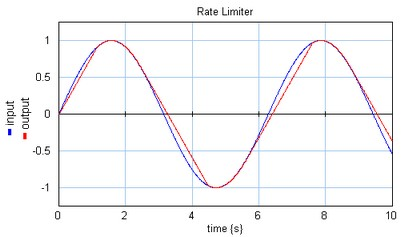﻿ 20-sim webhelp > Library > Signal > Block Diagram Non-Linear > signallimiter-ratelimit

# signallimiter-ratelimit

## Library

Signal\Block Diagram Non-Linear

## Use

Domains: Discrete, Continuous. Size: 1-D. Allowed in: Block Diagrams.

## Description

This model limits the input signal to a minimum and maximum rate.

doutput/dt = dinput/dt; (minimum < dinput/dt < maximum)

doutput/dt = minimum; (dinput/dt < minimum)

output = maximum (dinput/dt > maximum)

En example is shown below where a sinusoidal input signal is limited to a maximum rate of 0.4 and a minimum rate of -0.8.The rate limiter uses a first order filter to approximate the derivative of the input. Below the cut-off frequency fl the approximation is accurate and the model behaves as a rate limiter. Above the cut-off frequency fl the model output weakens to zero. For high values of the cut-off frequency fl, the model will slow down simulation because it forces the integration method to take very small time-steps. A good trade-off for most models is a starting value of 100 [Hz].

## Interface

 Inputs Description input Outputs output Parameters minimum maximum fl Minimum rate of the output. Maximum rate of the output. Cut-off frequency [Hz]